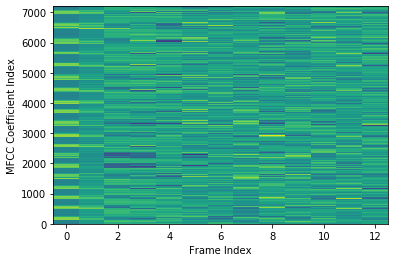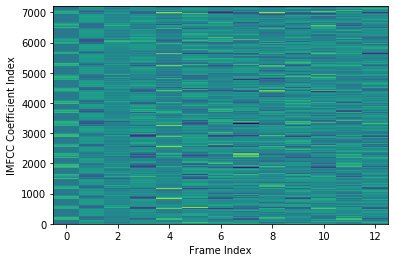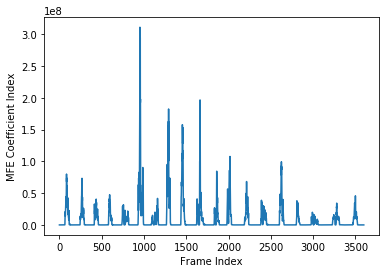# spafe.features.mfcc¶

spafe.features.mfcc.imfcc(sig, fs=16000, num_ceps=13, pre_emph=0, pre_emph_coeff=0.97, win_len=0.025, win_hop=0.01, win_type='hamming', nfilts=26, nfft=512, low_freq=None, high_freq=None, scale='constant', dct_type=2, use_energy=False, lifter=22, normalize=1)[source]

Compute Inverse MFCC features from an audio signal.

Parameters: sig (array) – a mono audio signal (Nx1) from which to compute features. fs (int) – the sampling frequency of the signal we are working with. Default is 16000. num_ceps (float) – number of cepstra to return. Default is 13. pre_emph (int) – apply pre-emphasis if 1. Default is 1. pre_emph_coeff (float) – apply pre-emphasis filter [1 -pre_emph] (0 = none). Default is 0.97. win_len (float) – window length in sec. Default is 0.025. win_hop (float) – step between successive windows in sec. Default is 0.01. win_type (float) – window type to apply for the windowing. Default is “hamming”. nfilts (int) – the number of filters in the filterbank. Default is 40. nfft (int) – number of FFT points. Default is 512. low_freq (int) – lowest band edge of mel filters (Hz). Default is 0. high_freq (int) – highest band edge of mel filters (Hz). Default is samplerate / 2 = 8000. scale (str) – choose if max bins amplitudes ascend, descend or are constant (=1). Default is “constant”. dct_type (int) – type of DCT used - 1 or 2 (or 3 for HTK or 4 for feac). Default is 2. use_energy (int) – overwrite C0 with true log energy Default is 0. lifter (int) – apply liftering if value > 0. Default is 22. normalize (int) – apply normalization if 1. Default is 0. features - the MFFC features: num_frames x num_ceps (array)
spafe.features.mfcc.mfcc(sig, fs=16000, num_ceps=13, pre_emph=0, pre_emph_coeff=0.97, win_len=0.025, win_hop=0.01, win_type='hamming', nfilts=26, nfft=512, low_freq=None, high_freq=None, scale='constant', dct_type=2, use_energy=False, lifter=22, normalize=1)[source]

Compute MFCC features (Mel-frequency cepstral coefficients) from an audio signal. This function offers multiple approaches to features extraction depending on the input parameters. Implemenation is using FFT and based on http://citeseerx.ist.psu.edu/viewdoc/download?doi=10.1.1.63.8029&rep=rep1&type=pdf

• take the absolute value of the FFT
• warp to a Mel frequency scale
• take the DCT of the log-Mel-spectrum
• return the first <num_ceps> components
Parameters: sig (array) – a mono audio signal (Nx1) from which to compute features. fs (int) – the sampling frequency of the signal we are working with. Default is 16000. num_ceps (float) – number of cepstra to return. Default is 13. pre_emph (int) – apply pre-emphasis if 1. Default is 1. pre_emph_coeff (float) – apply pre-emphasis filter [1 -pre_emph] (0 = none). Default is 0.97. win_len (float) – window length in sec. Default is 0.025. win_hop (float) – step between successive windows in sec. Default is 0.01. win_type (float) – window type to apply for the windowing. Default is “hamming”. nfilts (int) – the number of filters in the filterbank. Default is 40. nfft (int) – number of FFT points. Default is 512. low_freq (int) – lowest band edge of mel filters (Hz). Default is 0. high_freq (int) – highest band edge of mel filters (Hz). Default is samplerate / 2 = 8000. scale (str) – choose if max bins amplitudes ascend, descend or are constant (=1). Default is “constant”. dct_type (int) – type of DCT used - 1 or 2 (or 3 for HTK or 4 for feac). Default is 2. use_energy (int) – overwrite C0 with true log energy Default is 0. lifter (int) – apply liftering if value > 0. Default is 22. normalize (int) – apply normalization if 1. Default is 0. features - the MFFC features: num_frames x num_ceps (array)

Example:

import scipy.io.wavfile
import spafe.utils.vis as vis
from spafe.features.mfcc import mfcc, imfcc, mfe